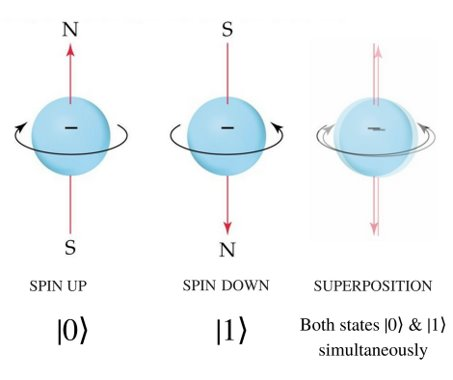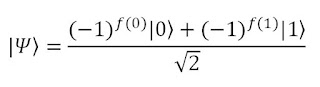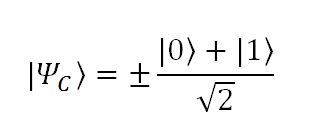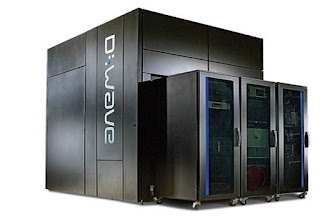# The Wacky World of Quantum Computing

You don’t have to be a computer scientist to know that computing power has been increasing dramatically over the past decades, bringing us ever smaller and faster devices. This is driven by our ability to make transistors, the fundamental element of the computer chip, smaller and smaller. It turns out, though, that there’s a limit to how small these can get before they fail due to the strange effects of quantum mechanics, and we are fast approaching it.

However, it turns out that the very physics that limit our computers may provide the solutions. These are the so-called quantum computers, which hold the promise of exponentially faster computers. To understand quantum computing, you first have to understand two fundamental principles of quantum physics: superposition and quantum entanglement. I’ll start with superposition first.

#### Superposition

In physics, as in the easily observable world, there are some quantities that can be thought of as discrete or mutually exclusive. One commonly-used example is that of a coin: if you flip a quarter, barring some freak act of nature, you’re guaranteed to get either heads or tails, with no other possibilities. In quantum physics it’s more convenient to talk about a property called electron spin, which is just a number describing a possible state of an electron: it’s either spin up or spin down. This sounds complicated, but you can think of it as analogous to the coin landing heads-up or heads-down.

Well, this holds quite nicely– until we get to the world of quantum phenomena. It turns out that according to quantum mechanics, an electron can have both spin up and spin down at the same time! This might not sound crazy if you aren’t used to working with electron spins, but if we extend this to the macroscopic level, that’s like saying that a single coin toss can result in both heads and tails– weird! This is what’s known as a superposition of states. It’s impossible to say whether the electron is spin-up or spin-down because it is effectively both.

So how do we know this? It’s tricky because if we try to measure an electron that’s in superposition our instrument isn’t going to read “spin up and spin down”. The world just doesn’t work like that (just like your speedometer won’t tell you that you’re going 45mph and 70mph at once). Instead it will just give us a normal readout: either spin up or spin down. Instead, we know about superposition through indirect observations.

So what happened to the superposition when we measured it? It turns out that this crazy state just collapsed into a regular spin-up or spin-down system, like you would expect before I introduced the idea of superposition at all. So that’s a key point: systems in superposition are extremely delicate and collapse upon measurement. What counts as a measurement? This is a hotly contested metaphysical idea, but essentially any time we gain information about the electron that counts.This is a representation of electron spin superposition. The symbols underneath use bra-ket notation to describe the states (so spin up is “ket-zero” and spin down is “ket-one”. We use this notation because computer theory is traditionally based on zeros and ones (binary) so this makes it easier to use existing structures to work out the theory of quantum computers.

Formally, we can describe superposition using the wave function:

All this means is that the wave function “psi” has a certain “amount” (given by alpha) of state zero and a certain amount (given by beta) of state one.

Now, there’s a lot you can do with a wave function but in the interest of brevity I’ll just say that when you measure the spin of an electron in this superposition, the likelihood of getting zero or one depends on the values of alpha and beta (the coefficients).

#### Entanglement

So great, I just showed you how to describe the state of one electron spin. But what happens if we have two (or more) electrons? What do their wave functions look like? It turns out that you can rewrite them pretty easily:

All this says is that if you measure both electron spins, you are bound to get two zeros, two ones, or some combination of one and zero; again, the likelihood of each possibility is dependent on the coefficients out front.

One thing that’s useful to note is that if you measure only one of the electron spins, you can update the wave function of the second electron based on the outcome. This effectively gives us a “guess” about what the second electron will look like when we measure it without actually collapsing its wave function. However, there’s an even niftier trick that allows us to know with certainty what a measurement of the second electron will yield– without actually measuring it. This is called entanglement. Take a look at this wave function:Notice that if we measure the first electron spin to be zero, the second spin must also be zero– there simply isn’t any allowance for a zero-one combination! The cool thing is that once two electrons are entangled like this, it doesn’t matter what you do to them or how far away you take them from each other, their wave functions are linked. By using clever manipulations, we’re able to take advantage of this (as I’ll show later on).

OK, so now that we have the basics of quantum physics out of the way it should be pretty smooth sailing (well, sort of). How does do superposition and entanglement fit into the world of computing? Well may you ask.

#### Qubits

You may know that classical computers are essentially a bunch of binary digits (“bits”) that can take on the state zero or one. In concrete terms, this typically means high or low voltage, although you can really make a computer out of anything. By performing gate operations on these bits, we can manipulate data and get some sort of useful result. Generally speaking, this works fairly well, but there’s a limit to how good computers can get; it turns out that as we’re making transistors smaller and smaller we start to run into quantum effects that make them useless, and the best supercomputers in the world are still unable to run certain types of simulations. What this really comes down to is the fact that a bit can take on the value zero or one, but never both, so if we want to run a computation on zero and then the same computation on one, we have to do it twice– not very efficient! What if, on the other hand, we had some sort of quantum bit (or “qubit”) that could take on the state zero and one, so that we only had to perform the computation once? You may see where this is going…

#### Gate Manipulation

Sure enough, if we had quantum bits rather than regular bits our computing power would increase exponentially. In fact, it would only take a 50-qubit computer to outperform every computer ever created so far! The trick is actually making these qubits; you may remember that I mentioned how delicate a superposition state is, and the power of the qubit is in its superposition. So if any sort of interaction between a qubit and the outer world causes the wave function to collapse, well, we’re just dealing with a regular computer now. Although researchers are still working to develop effective quantum computers, the mathematical theory behind them is well understood. Essentially, we already have quantum algorithms and programs, just no computer to run them on.

All quantum gates are mathematically represented by a unitary matrix, which is just a special class of matrix. Then if we represent the wave function in matrix formMathematically we can consider the gate transformation to be simple matrix multiplication. That’s a lot to think about, so here’s a concrete example:So when the initial wave function passes through the gate (given by the funky matrix) you see that the “amounts” of zero and one are reversed. In classical terms, if we had a zero we’d get a one and if we had a one we’d get a zero; this is just the quantum version of a NOT gate!

#### A Concrete Example

This is great, but how can it help us solve problems quicker? To take a very silly example, let’s look at the case of constant and balanced functions. In Boolean algebra (the world of zeros and ones) a function is considered constant if its output doesn’t depend on input (just like in regular math) and balanced otherwise. If we have a function f that acts as a black box, to figure out whether it’s constant or balanced on a classical computer we’d need to test both cases (first plug in a zero, then a one) to decide what type of function f is.

But– what if we use a quantum computer? Let’s say we have a way of preparing a wave function (we do) that looks like this:It’s ugly, but it turns out that if f is a constant function the wave function becomesConversely, if it’s balanced the wave function isSo now that we have two distinct possibilities for the wave function of our qubit, depending on whether f is constant or balanced, all we need is to find a way to distinguish between the two. It turns out that a Hadamard gate will do just the trick:It looks messy, but the meaning is beautifully simple: if we start with a balanced function, upon measuring the output we will always get state one, with 100% certainty. Similarly, if we had started with a constant function, we would get state zero. Essentially, we’ve done with one computation what would have taken two on a classical computer.

Mind you, this is a trivial example and doesn’t save much work (as a matter of fact, it may not save any since we first have to prepare the wave function properly). However, it gives you a sense of how powerful this technique could be scaled up.

#### Quantum Computers in Practice

All of this is great, but what does it mean outside of the realm of math and computer science? Well, there are a ton of labs working on just this. Notice that qubits– just like classical bits– could theoretically be made out of anything, as long as it can exist in a superposition. Some popular choices include electron spin (like in my superposition example) and the polarization of light. The critical thing is that the qubits must be able to entangle with each other (so that we can manipulate them and make measurements without completely collapsing the wave function) and not with the environment– and it turns out that this is very tricky.

One exciting project comes from the University of New South Wales, which is developing some promising silicon qubits. This is a huge plus since practically all current computers are silicon-based, so if we could adapt the existing infrastructure rather than starting over from scratch we’d save tons of money (and effort). Their most recent development is a single phosphorous atom embedded in silicon with an electrode placed above. In this case, the states are given by the spin of a single electron and the neutron, and the qubit can be manipulated using the electrodes. More importantly, the electrodes cause the atom to “stretch” a bit as the electrons move closer to the surface, creating an electric field. It turns out that this field is key to allowing the qubits to entangle with each other at appreciable distances.This is an artist’s representation of the NSW team’s qubit system.

Finally, I should note that there are machines currently being marketed as quantum computers; for just \$15 million you too could own a D-Wave machine!  Although they’ve had some success– and high-profile customers– there’s a lot of debate about whether the D-Wave can really be considered a true quantum computer. That’s because it uses not the gate-based manipulation I’ve just described but a totally different process called reverse annealing to perform its calculations. If the system is set up just right to reflect a given problem (typically optimization or sampling related) and then allowed to evolve, it will tend to settle in the lowest-energy states, thus providing an answer of some form. This works well for some types of problems, but fails to harness the true power of quantum computers.